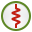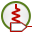# LT Card

This card assigns a resistor, inductor or capacitor in series to a voxel mesh for the finite difference time domain (FDTD) method.

On the Source/Load tab, in the Loads / networks group, click theLoad icon. From the drop-down list, click thePort load (LT) icon.

## Parameters:

Define/replace a load at the given port
Define a load or replace a load at the given port with the parameters specified below.
Remove all LT type loads previously defined
This LT card does not define a load, but rather all previously defined LT loads are deleted. All the other input parameters of this card are ignored.
Name of port definition
The name of the port.
Discrete element
A single resistor, inductor or capacitor is added to the port.
Resistor
Value of the resistor in $\Omega$ .
Inductor
Value of the inductor in H.
Capacitor
Value of the capacitor in F.
Complex impedance
The real and imaginary part of the complex impedance in $\Omega$ .
Series circuit
The resistor value in $\Omega$ , inductor value in Henry and the capacitor value in Farad to be added as a series circuit.
Parallel circuit
The resistor value in $\Omega$ , inductor value in Henry and the capacitor value in Farad to be added as a parallel circuit.
Resistor value (Ohm)
Value of the resistor in $\Omega$ .